One of my favorite magazines, Significance, printed an intriguing image of a symmetric matrix that shows repetition in a song's lyrics. The image was created by Colin Morris, who has created many similar images. When I saw these images, I knew that I wanted to duplicate the analysis in SAS!

### Visualize repetition in lyrics: A simple example

The analysis is easy. Suppose that a song (or any text source) contains N words. Define the repetition matrix to be the N x N matrix where the (i,j)th cell has the value 1 if the i_th word is the same as the j_th word. Otherwise, the (i,j)th cell equals 0. Now visualize the matrix by using a heat map: Black indicates cells where the matrix is 1 and white for 0. A SAS program that performs this analysis is available at the end of this article.

To illustrate this algorithm, consider the nursery rhyme, "Row, Row, Row the Boat":

```Row, row, row your boat Gently down the stream Merrily, merrily, merrily, merrily Life is but a dream.```

There are 18 words in this song. Words 1–3 are repeated, as are words 1-–13. You can use the SAS DATA steps to read the words of the song into a variable and use other SAS functions to strip out any punctuation. You can then use SAS/IML software to construct and visualize the repetition matrix. The details are shown at the end of this article.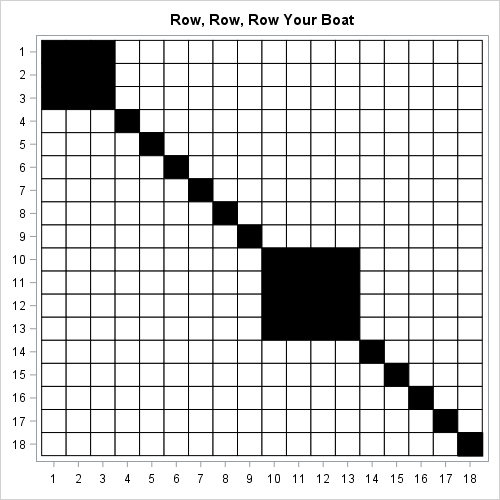The repetition matrix for the song "Row, Row, Row, Your Boat" is shown to the right. For this example I could have put the actual words along the side and bottom of the matrix, but that is not feasible for songs that have hundreds of words. Instead, the matrix has a numerical axis where the number indicates the position of each word in the song.

Every repetition matrix has 1s on the diagonal. In this song, the words "row" and "merrily" are repeated. Consequently, there is a 3 x 3 block of 1s at the top left and a 4 x 4 block of 1s in the middle of the matrix. (Click to enlarge.)

As mentioned, this song has very little repetition. One way to quantify the amount of repetition is to compute the proportion of 1s in the upper triangular portion of the repetition matrix. The upper triangular portion of an N x N matrix has N(N–1)/2 elements. For this song, N=18, so there are 153 cells and 9 of them are 1s. Therefore the "repetition score" is 9 / 153 = 0.059.

### Another simple example of repetition in song lyrics

I wrote a SAS/IML function that creates and visualizes the repetition matrix and returns the repetition score. In order to visualize songs that might have hundreds of words, I suppress the outlines (the grid) in the heat map. To illustrate the output of the function, the following image visualizes the words of the song "Here We Go Round the Mulberry Bush":

```Here we go round the mulberry bush, The mulberry bush, The mulberry bush. Here we go round the mulberry bush So early in the morning.```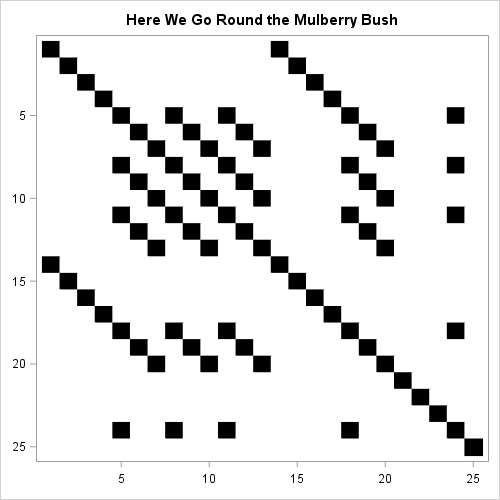The repetition score for this song is 0.087. You can see diagonal "stripes" that correspond to the repeating phrases "here we go round" and "the mulberry bush". In fact, if you study only the first seven rows, you can "see" almost the entire structure of the song. The first seven words contain all lyrics except for four words ("so", "early", "in", "morning").

### Visualize Classic Song Lyrics

Let's visualize the repetitions in the lyrics of several classic songs.

#### Hey Jude (The Beatles)

When I saw Morris's examples, the first song I wanted to visualize was "Hey Jude" by the Beatles. Not only does the title phrase repeat throughout the song, but the final chorus ("Nah nah nah nah nah nah, nah nah nah, hey Jude") repeats more than a dozen times. This results in a very dense block in the lower right corner of the repetition matrix and a very high repetition score of 0.183. The following image visualizes "Hey Jude":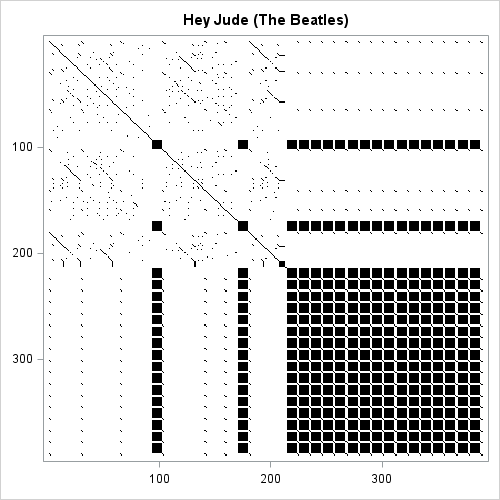#### Love Shack (The B-52s)

The second song that I wanted to visualize was "Love Shack" by The B-52s. In addition to a title that repeats almost 40 times, the song contains a sequence near the end in which the phrase "Bang bang bang on the door baby" is alternated with various interjections. The following visualization of the repetition matrix indicates that there is a lot of variation interspersed with regular repetition. The repetition score is 0.035.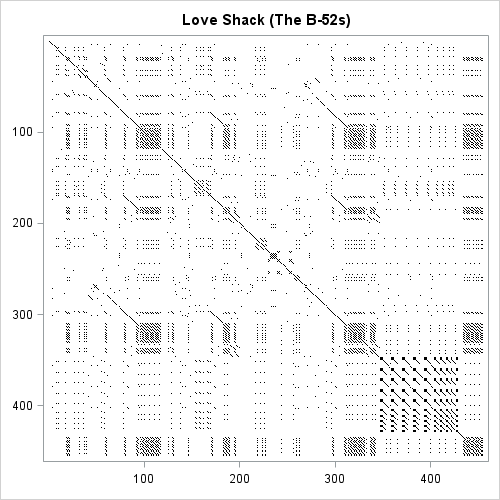#### Call Me (Blondie)

Lastly, I wanted to visualize the song "Call Me" by Blondie. This classic song has only 241 words, yet the title is repeated 41 times! In other words, about 1/3 of the song consists of those two words! Furthermore, there is a bridge in the middle of the song in which the phrase "oh oh oh oh oh" is alternated with other phrases (some in Italian and French) that appear only once in the song. The repetition score is 0.077. The song is visualized below: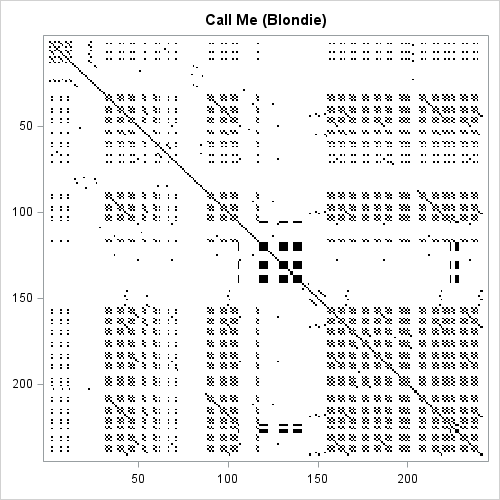### How to create a repetition matrix in SAS

If you think this is a fun topic, you can construct these images yourself by using SAS. If you discover a song that has an interesting repetition matrix, post a comment!

Here's the basic idea of how to construct and visualize a repetition matrix. First, use the DATA step to read each word, use the COMPRESS function to remove any punctuation, and standardize the input by transforming all words to lowercase:
```data Lyrics; length word \$20; input word @@; word = lowcase( compress(word, ,'ps') ); /* remove punctuation and spaces */ datalines; Here we go round the mulberry bush, The mulberry bush, The mulberry bush. Here we go round the mulberry bush So early in the morning. ;```

In SAS/IML software you can use the ELEMENT function to find the locations in the i_th row that have the value 1. After you construct a repetition matrix, you can use the HEATMAPDISC subroutine to display it. For example, the following SAS/IML program reads the words of the song into a vector and visualizes the repetition matrix. It also returns the repetition score, which is the proportion of 1s in the upper triangular portion of the matrix.

```ods graphics / width=500 height=500 NXYBINSMAX=1000000; proc iml; /* define a function that creates and visualizes the repetition matrix */ start VizLyrics(DSName, Title); use (DSName); read all var _CHAR_ into Word; close; N = nrow(Word); M = j(N,N,0); /* allocate N x N matrix */ do i = 1 to N; M[,i] = element(Word, Word[i]); /* construct i_th row */ end; run heatmapdisc(M) title=Title colorramp={white black} displayoutlines=0 showlegend=0;   /* compute the proportion of 1s in the upper triangular portion of the matrix */ upperIdx = loc(col(M)>row(M)); return ( M[upperIdx][:] ); /* proportion of words that are repeated */ finish;   score = VizLyrics("Lyrics", "Here We Go Round the Mulberry Bush"); print score;```

If you want to reproduce the images in this post, you can download the SAS program for this article. In addition, the program creates repetition matrices for "We Didn't Start the Fire" (Billy Joel) and a portion of Martin Luthor King Jr.'s "I Have a Dream" speech. You can modify the program and enter lyrics for your favorite songs.

The post Visualize repetition in song lyrics appeared first on The DO Loop.When I first learned to program in SAS, I remember being confused about the difference between CLASS statements and BY statements. A novice SAS programmer recently asked when to use one instead of the other, so this article explains the difference between the CLASS statement and BY variables in SAS procedures.

The BY statement and the CLASS statement in SAS both enable you to specify one or more categorical variables whose levels define subgroups of the data. (For simplicity, we consider only a single categorical variable.) The primary difference is that the BY statement computes many analyses, each on a subset of the data, whereas the CLASS statement computes a single analysis of all the data. Specifically,

• The BY statement repeats an analysis on every subgroup. The subgroups are treated as independent samples. If a BY variable defines k groups, the output will contains k copies of every table and graph, one copy for the first group, one copy for the second group, and so on.
• The CLASS statement enables you to include a categorical variable as part of an analysis. Often the CLASS variable is used to compare the groups, such as in a t test or an ANOVA analysis. In regression models, the CLASS statement enables you to estimate parameters for the levels of a categorical variable, thereby estimating the effect of each level on the response. Another use of a CLASS variable is to define categories for a classification task, such as a discriminant analysis.

To illustrate the differences between an analysis that uses a BY statement and one that uses a CLASS statement, let's create a subset (called Cars) of the Sashelp.Cars data. The levels of the Origin variable indicate whether a vehicle is manufactured in "Asia", "Europe", or the "USA". For efficiency reasons, most classical SAS procedures require that you sort the data when you use a BY statement. Therefore, a call to PROC SORT creates a sorted version of the data called CarsSorted, which will be used for the BY-group analyses.

```data Cars; set Sashelp.Cars; where cylinders in (4,6,8) and type ^= 'Hybrid'; run;   proc sort data=Cars out=CarsSorted; by Origin; run;```

### Descriptive statistics for grouped data

When you generate descriptive statistics for groups of data, the univariate statistics are identical whether you use a CLASS statement or a BY statement. What changes is the way that the statistics are displayed. When you use the CLASS statement, you get one table that contains all statistics or one graph that shows the distribution of each subgroup. However, when you use the BY statement you get multiple tables and graphs.

The following statements use the CLASS statement to produce descriptive statistics. PROC UNIVARIATE displays one (paneled) graph that shows a comparative histogram for the vehicles that are made in Asia, Europe, and USA. PROC MEANS displays one table that contains descriptive statistics:

```proc univariate data=Cars; class Origin; var Horsepower; histogram Horsepower / nrows=3; /* must use NROWS= to get panel */ ods select histogram; run;   proc means data=Cars N Mean Std; class Origin; var Horsepower Weight Mpg_Highway; run;```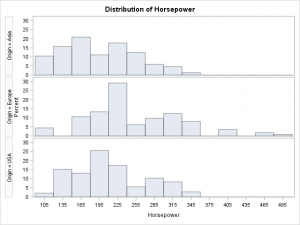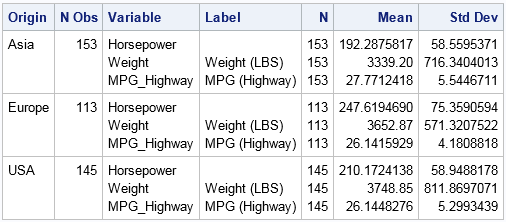In contrast, if you run a BY-group analysis on the levels of the Origin variable, you will see three times as many tables and graphs. Each analysis is preceded by a label that identifies each BY group. Notice that the BY-group analysis uses the sorted data.

```proc means data=CarsSorted N Mean Std; by Origin; var Horsepower Weight Mpg_Highway; run;```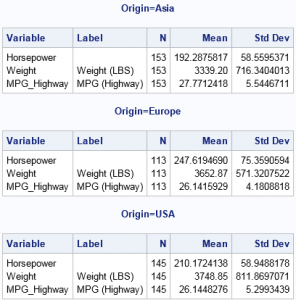Always remember that the output from a BY statement is equivalent to the output from running the procedure multiple times on subsets of the data. For example, the previous statistics could also be generated by calling PROC MEANS three times, each call with a different WHERE clause, as follows:

```proc means N Mean Std data=CarsSorted( where=(origin='Asia') ); var Horsepower Weight Mpg_Highway; run; proc means N Mean Std data=CarsSorted( where=(origin='Europe') ); var Horsepower Weight Mpg_Highway; run; proc means N Mean Std data=CarsSorted( where=(origin='USA') ); var Horsepower Weight Mpg_Highway; run;```

In fact, if you ever find yourself repeating an analysis many times (perhaps by using a macro loop), you should consider whether you can rewrite your program to be more efficient by using a BY statement.

### Comparing groups: Use the CLASS statement

As a general rule, you should use a CLASS statement when you want to compare or contrast groups. For example, the following call to PROC GLM performs an ANOVA analysis on the horsepower (response variable) for the three groups defined by the Origin variable. The procedure automatically creates a graph that displays three boxplots, one for each group. The procedure also computes parameter estimates for the levels of the CLASS variable (not shown).

```proc glm data=Cars; /* by default, create graph with side-by-side boxplots */ class Origin; model Horsepower = Origin / solution; run;```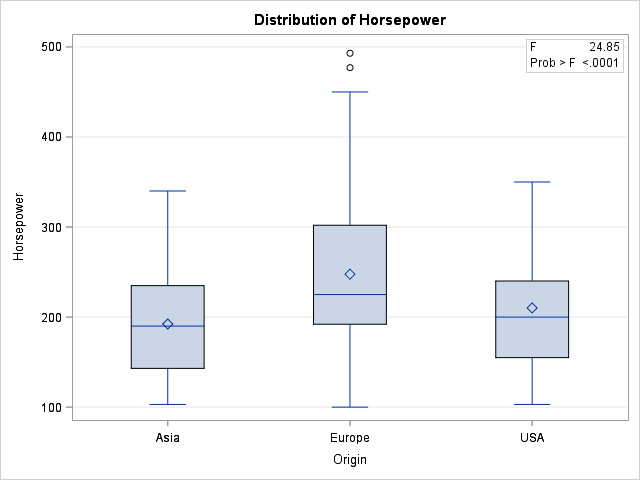You can specify multiple variables on the CLASS statement to include multiple categorical variables in a model. Any variables that are not listed on the CLASS statement are assumed to be continuous. Thus the following call to PROC GLM analyzes a model that has one continuous and one classification variable. The procedure automatically produces a graph that overlays the three regression curves on the data:

```ods graphics /antialias=on; title "CLASS Variable Regression: One Model with Multiple Parameters"; proc GLM data=Cars plots=FitPlot; class Origin; model Horsepower = Origin | Weight / solution; ods select ParameterEstimates ANCOVAPlot; quit;```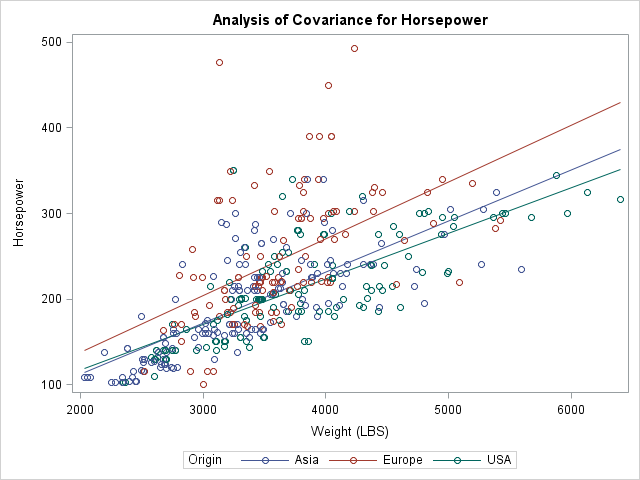In contrast, if you use a BY statement, the Origin variable cannot be part of the model but is used only to subset the data. If you use a BY statement, you obtain three different models of the form Horsepower = Weight. You get three parameter estimates tables and three graphs, each showing one regression line overlaid on a subset of the data.

### Predicted Values: CLASS VARIABLE versus BY Variable

When you use a BY statement and fit three models of the form Horsepower = Weight, the procedure fits a total of six parameters. Notice that when you use the CLASS statement and fit the model Horsepower = Origin | Weight, you also fit six free parameters. It turns out that these two methods produce the same predicted values. In fact, you can combine the parameter estimates (for the GLM parameterization) for the CLASS model to obtain the parameter estimates from the BY-variable analysis, as shown below. Each parameter estimate for the BY-variable models are obtained as the sum of two estimates for the CLASS-variable analysis: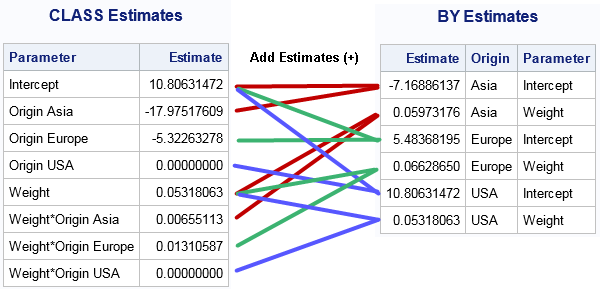For many regression models, the predicted values for the BY-variable analyses are the same as for a particular model that uses a CLASS variable. As shown above, you can even see how the parameters are related when you use a GLM or reference parameterization. However, the CLASS variable formulation can fit models (such as the equal-slope model Horsepower = Origin Weight) that are not available when you use a BY variable to fit three separate models. Furthermore, the CLASS statement provides parameter estimates so that you can see the effect of the groups on the response variable. It is more difficult to compare the models that are produced by using the BY statement.

### Other CLASS-like statements in SAS

Some SAS procedures use other syntax to analyze groups. In particular, the SGPLOT procedure calls classification variables "group variables." If you want to overlay graphs for multiple groups, you can use the GROUP= option on many SGPLOT statements. (Some statements support the CATEGORY= option, which is similar.) For example, to replicate the two-variable regression analysis from PROC GLM, you can use the following statements in PROC SGPLOT:

```proc sgplot data=Cars; reg y=Horsepower x=Weight / group=Origin; /* Horsepower = Origin | Weight */ run;```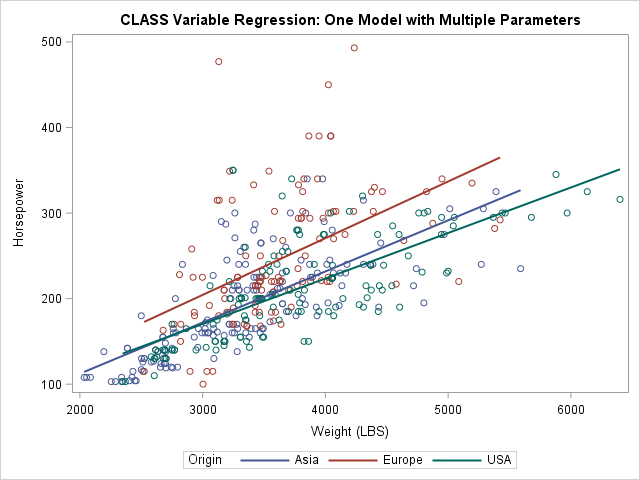### Summary

In summary, use the BY statement in SAS procedures when you want to repeat an analysis for every level of one or more categorical variables. The variables define the subsets but are not otherwise part of the analysis. In classical SAS procedures, the data must be sorted by the BY variables. A BY-group analysis can produce many tables and graphs, so you might want to suppress the ODS output and write the results to a SAS data set.

Use the CLASS statement when you want to include a categorical variable in a model. A CLASS statement often enables you to compare or contrast subgroups. For example, in regression models you can evaluate the relative effect of each level on the response variable.

In some cases, the BY statement and the CLASS statement produce identical statistics. However, the CLASS statement enables you to fit a wider variety of models.

The post The difference between CLASS statements and BY statements in SAS appeared first on The DO Loop.Last week I wrote about the 10 most popular articles from The DO Loop in 2017. My most popular articles tend to be about elementary statistics or SAS programming tips. Less popular are the articles about advanced statistical and programming techniques. However, these technical articles fill an important niche. Not everyone needs to know how to interpret a diffogram that visually compares the differences between means between groups, but those who do often send me a note of thanks, which motivates me to research and write similar articles.

Statistical programmers might want to review the following technical articles from 2017. This ain't summertime, and the reading ain't easy, but I think these articles are worth the effort. I've broken them into three categories. Enjoy!

### Statistical Concepts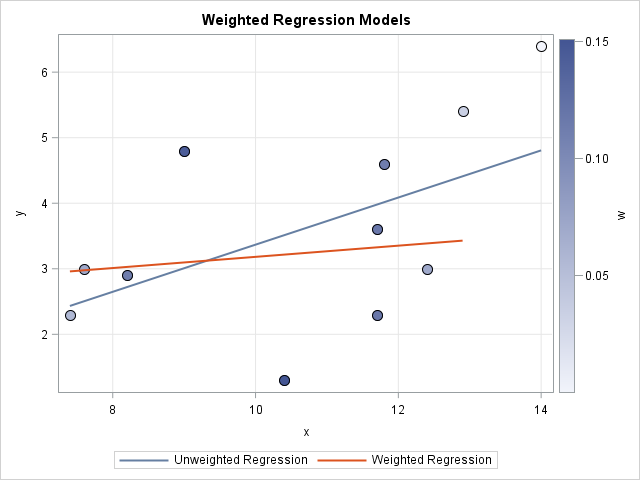### Statistical Data Analysis and Visualization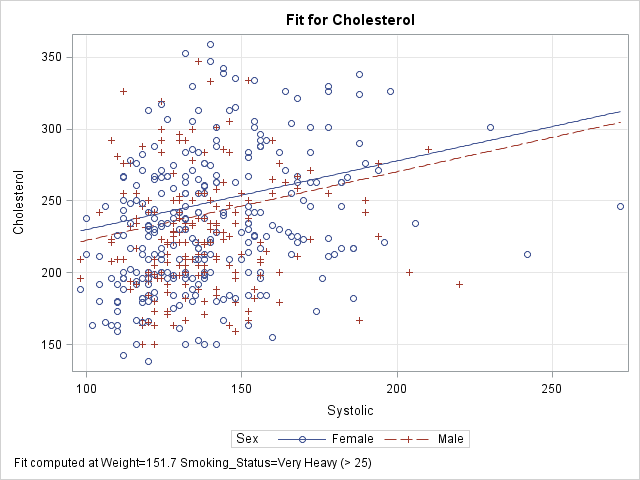### Advanced SAS Programming Techniques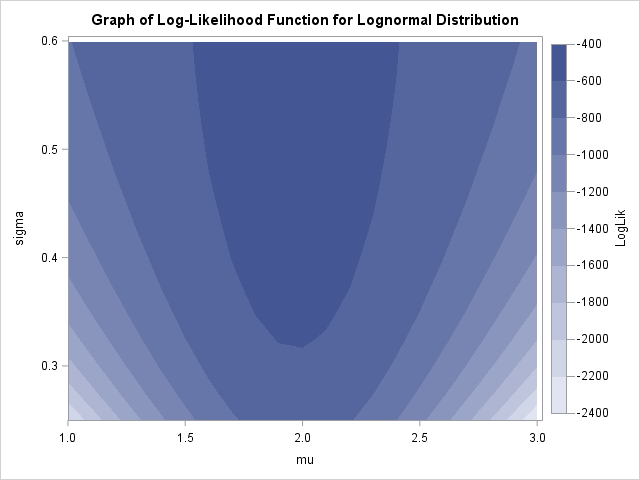If you are searching for a way to enhance your SAS knowledge in this New Year, I think these 10 articles from The DO Loop are worth a second look. Was there an article from 2017 that you found particularly useful? Leave a comment.

The post 10 posts from 2017 that deserve a second look appeared first on The DO Loop.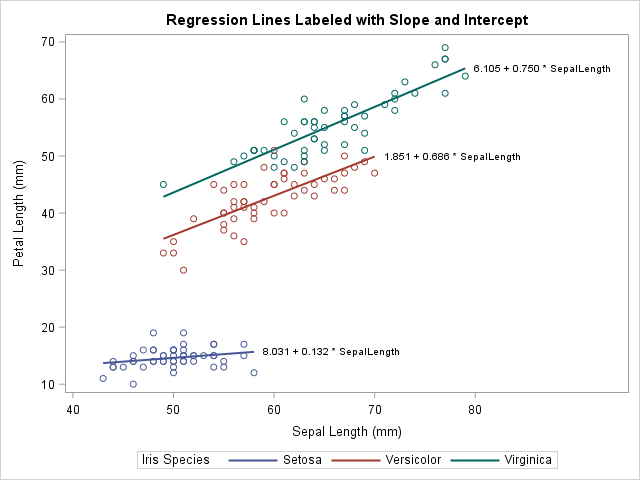A SAS programmer asked how to label multiple regression lines that are overlaid on a single scatter plot. Specifically, he asked to label the curves that are produced by using the REG statement with the GROUP= option in PROC SGPLOT. He wanted the labels to be the slope and intercept of a linear regression line, as shown to the right. (Click to enlarge.)

Initially I thought that you could use the CURVELABEL option on the REG statement to generate labels, as follows:

```proc sgplot data=sashelp.iris noautolegend; reg x=SepalLength y=PetalLength / group=Species CURVELABEL; /* does NOT work */ run;```

However, the SAS log displays the following warning:

```WARNING: CURVELABEL not supported for fit plots when a group variable is used. The option will be ignored.```

Fortunately, I thought of two other ways to create a graph that has a regression line for each group level, each with its own label. For linear regression, you can use the LINEPARM statement, as shown in the article "Add a diagonal line to a scatter plot." For general (possibly nonlinear) regression curves, you can find the location of the end of the curve and use the TEXT statement in PROC SGPLOT to add a label at that location.

### Label the regression line for each group: The LINEPARM statement

Let's use Fisher's Iris data set for our example data. The Iris data contains 50 observations for each of three species of flowers: iris Setosa, iris Versicolor, and iris Virginica. The programmer wants to label the regression line for each species by using the slope and intercept of the line. The first step is to create a SAS data set that contains the intercept and slope for each curve. You can use the OUTEST= option in PROC REG to write the parameter estimates (intercept and slope) to a SAS data set. You can then use the CATX function in the DATA step to construct the labels, as follows:

```proc sort data=sashelp.iris out=iris; by Species; run;   /* compute parameter estimates */ proc reg data=iris outest=PE noprint; by Species; model PetalLength = SepalLength; run;   /* construct labels from the parameter estimates */ data Labels; length Label \$30; set PE(rename=(SepalLength=Slope)); /* independent variable */ Label = catx(" ", put(Intercept, BestD5.), '+', /* separate by blank */ put(Slope, BestD5.), '* SepalLength'); keep Label Species Intercept Slope; run;   proc print noobs; run;```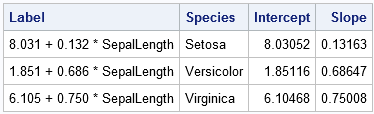The LABELS data set contains a label for the regression line in each group. You can use other labels if you prefer. The following DATA step combines the labels with the original data. The SCATTER statement in PROC SGPLOT displays the data. The LINEPARM statement draws the lines and adds labels to the end of each line.

```data Plot; set iris Labels; run;   title "Regression Lines Labeled with Slope and Intercept"; proc sgplot data=Plot; scatter x=SepalLength y=PetalLength / group=Species; lineparm x=0 y=Intercept slope=Slope / group=Label curvelabel curvelabelloc=outside clip; run;```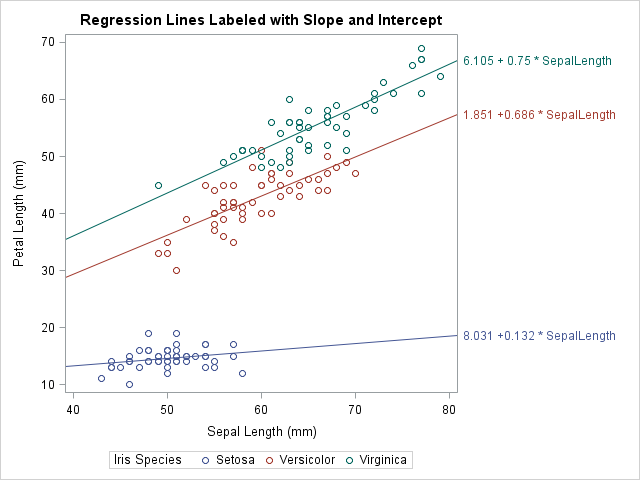Success! The regression line for each group is labeled by the formula for the line. For more information about displaying the formula for a regression line, see the SAS/STAT example "Adding Equations and Special Characters to Fit Plots."

### Label the regression line for each group: The TEXT statement

The preceding method uses the LINEPARM statement, so it only works for lines. However, the user actually wanted to use the REG statement. With a little work, you can label curves that are produced by the REG statement or other curve-fitting statements. The idea is to obtain the data coordinates for the end of the curve, which will become the location of the label.

You might be thinking, if the curve is produced by a regression statement in PROC SGPLOT, how can we get the data coordinates out of the plot and into a data set? The answer is simple: You can use the ODS OUTPUT statement to write a data set that contains the data in any ODS graph. You can apply this trick to any ODS graph, including graphs created by SGPLOT. The following call to PROC SGPLOT uses an ODS OUTPUT statement to create a SAS data set that contains the data in the regression plot:

```/* use ODS OUTPUT to find data coordinates of end of lines */ proc sgplot data=iris; ods output sgplot=RegPlot; /* name of ODS table is 'sgplot' */ reg x=SepalLength y=PetalLength / group=Species; run;   proc contents short varnum; run; /* find names used by graph */```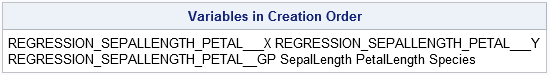The variable names that are automatically manufactured by SAS procedures can be long and unwieldy, as shown by the call to PROC CONTENTS. I usually rename the long names to simpler names such as X, Y, GROUP, and so on. You should look at the structure of the REGPLOT data set so that the next DATA step makes sense. The DATA step saves only the last coordinates along the curve for each group (species).

```data Coords; set RegPlot( rename=(REGRESSION_SEPALLENGTH_PETAL___X = x REGRESSION_SEPALLENGTH_PETAL___Y = y REGRESSION_SEPALLENGTH_PETAL__GP = Group) where=(x ^=.)); by Group; if last.Group; keep x y Group; run;   proc print noobs; run;```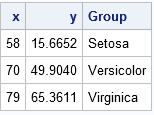The COORDS data contains the location (in data coordinates) of the end of each regression line. You can overlay labels at these coordinates to label the curves. From the preceding section, the labels are in the LABELS data set, so you can merge the two data sets, as follows:

```/* combine the positions and labels with original data */ data A; merge Labels Coords(rename=(Group=Species)); by Species; run;   data Plot; set iris A; /* optional: pad label with blanks on the left (if length is long enough) */ Label = " " || Label; run;   proc sgplot data=Plot; reg x=SepalLength y=PetalLength / group=Species; text x=x y=y text=Label / position=right; run;```

The graph is shown at the top of this article.

### Label multiple regression curves

If you study the previous section, you will see that the code does not rely on the linearity of the regression model. The same code works for polynomial regression and nonparametric regression curves such as are created by the LOESS and PBSPLINE statements in PROC SGPLOT. The following graph shows a PBSPLINE fit to the IRIS data. Because the penalized B-spline curve is nonparametric, there is no equation to display as a label. Instead, I use the Species name as a label and suppress the legend at the bottom of the graph. You can download the SAS program that creates this and all the graphs in this article.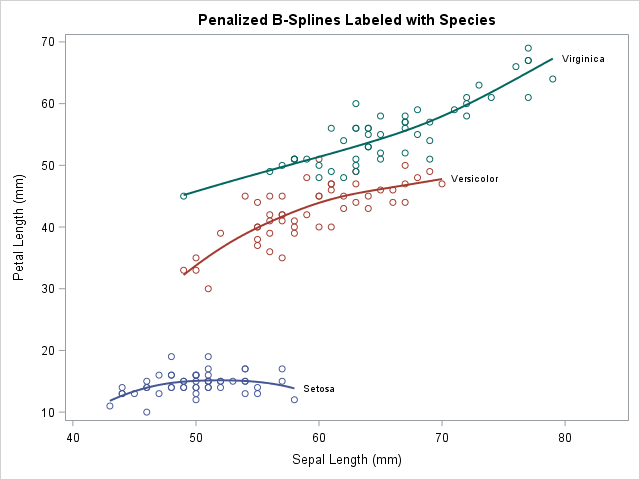The post Label multiple regression lines in SAS appeared first on The DO Loop.I wrote more than 100 posts for The DO Loop blog in 2017. The most popular articles were about SAS programming tips, statistical data analysis, and simulation and bootstrap methods. Here are the most popular articles from 2017 in each category.

### Statistics and Data Analysis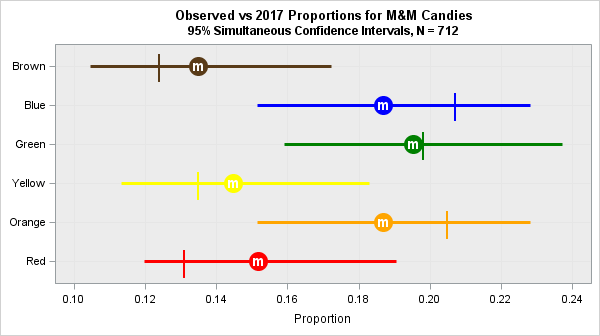• M&M Colors: It's no surprise that a statistical analysis of the color distribution of M&M candies was one of the most popular articles. Some people are content to know that the candies are delicious, but thousands wanted to read about whether blue and orange candies occur more often than brown.
• Interpretation of Correlation: Correlation is one of the simplest multivariate statistics, but it can be interpreted in many ways: algebraic, geometric, in terms of regression, and more. This article describes seven ways to view correlation?
• Winsorize Data: Before you ask "how can I Winsorize data" to eliminate outliers, you should ask "what is Winsorization" and "what are the pitfalls?" This article presents the advantages and disadvantages of Winsorizing data.

### Simulation and Bootstrapping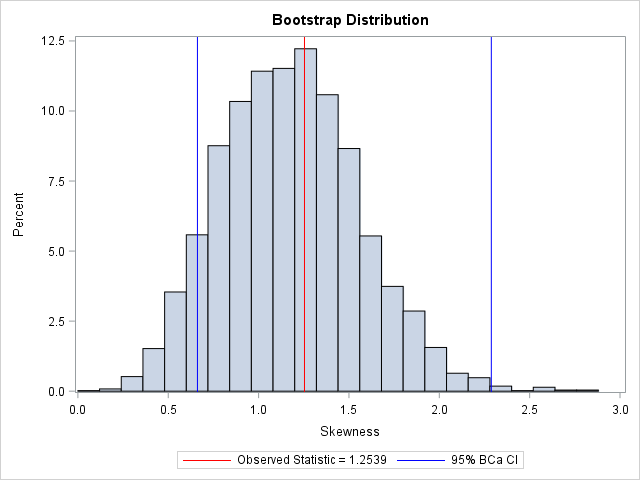Was you New Year's resolution to learn more about SAS? Did you miss any of these popular posts? Take a moment to read (or re-read!) one of these top 10 posts from the past year.

The post The top 10 posts from <em>The DO Loop</em> in 2017 appeared first on The DO Loop.I previously showed an easy way to visualize a regression model that has several continuous explanatory variables: use the SLICEFIT option in the EFFECTPLOT statement in SAS to create a sliced fit plot. The EFFECTPLOT statement is directly supported by the syntax of the GENMOD, LOGISTIC, and ORTHOREG procedures in SAS/STAT. If you are using another SAS regression procedure, you can still visualize multivariate regression models:

• If a procedure supports the STORE statement, you can save the model to an item store and then use the EFFECTPLOT statement in PROC PLM to create a sliced fit plot.
• If a procedure does not support the STORE statement, you can manually create the "slice" of observations and score the model on the slice.

### Use PROC PLM to score regression models

Most parametric regression procedures in SAS (GLM, GLIMMIX, MIXED, ...) support the STORE statement, which enables you to save a representation of the model in a SAS item store. The following program creates sample data for 500 patients in a medical study. The call to PROC GLM fits a linear regression model that predicts the level of cholesterol from five explanatory variables. The STORE statement saves the model to an item store named 'GLMModel'. The call to PROC PLM creates a sliced fit plot that shows the predicted values versus the systolic blood pressure for males and females in the study. The explanatory variables that are not shown in the plot are set to reference values by using the AT option in the EFFECTPLOT statement:

```data Heart; /* create example data */ set sashelp.heart(obs=500); where cholesterol < 400; run;   proc glm data=Heart; class Sex Smoking_Status BP_Status; model Cholesterol = Sex Smoking_Status BP_Status /* class vars */ Systolic Weight; /* contin vars */ store GLMModel; /* save the model to an item store */ run;   proc plm restore=GLMModel; /* load the saved model */ effectplot slicefit / at(Smoking_Status='Non-smoker' BP_Status='Normal' Weight=150); /* create the sliced fit plot */ run;```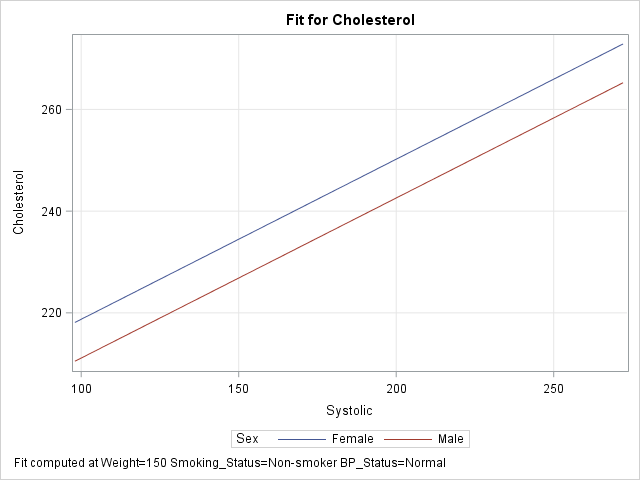The graph shows a sliced fit plot. The footnote states that the lines obtained by slicing through two response surfaces that correspond to (Smoking_Status, BP_Status) = ('Non-smoker', 'Normal') at the value Weight = 150. As shown in the previous article, you can specify multiple values within the AT option to obtain a panel of sliced fit plots.

### Create a sliced fit plot manually by using the SCORE statement

The nonparametric regression procedures in SAS (ADAPTIVEREG, GAMPL, LOESS, ...) do not support the STORE statement. Nevertheless, you can create a sliced fit plot using a traditional scoring technique: use the DATA step to create observations in the plane of the slice and score the model on those observations.

There are two ways to score regression models in SAS. The easiest way is to use PROC SCORE, the SCORE statement, or the CODE statement. The following DATA step creates the same "slice" through the space of explanatory variables as was created by using the EFFECTPLOT statement in the previous example. The SCORE statement in the ADAPTIVEREG procedure then fits the model and scores it on the slice. (Technical note: By default, PROC ADAPTIVEREG uses variable selection techniques. For easier comparison with the model from PROC GLM, I used the KEEP= option on the MODEL statement to force the procedure to keep all variables in the model.)

```/* create the scoring observations that define the slice */ data Score; length Sex \$6 Smoking_Status \$17 BP_Status \$7; /* same as for data */ Cholesterol = .; /* set response variable to missing */ Smoking_Status='Non-smoker'; /* set reference levels ("slices") */ BP_Status='Normal'; /* for class vars */ Weight=150; /* and continuous covariates */ do Sex = "Female", "Male"; /* primary class var */ do Systolic = 98 to 272 by 2; /* evenly spaced points for X variable */ output; end; end; run;   proc adaptivereg data=Heart; class Sex Smoking_Status BP_Status; model Cholesterol = Sex Smoking_Status BP_Status Systolic Weight / nomiss /* for comparison with other models, FORCE all variables to be selected */ keep=(Sex Smoking_Status BP_Status Systolic Weight); score data=Score out=ScoreOut Pred; /* score the model on the slice */ run;   proc sgplot data=ScoreOut; series x=Systolic y=Pred / group=Sex; /* create sliced fit plot */ xaxis grid; yaxis grid; run;```

The output, which is not shown, is very similar to the graph in the previous section.

### Create a sliced fit plot manually by using the missing value trick

If your regression procedure does not support a SCORE statement, an alternative way to score a model is to use "the missing value trick," which requires appending the scoring data set to the end of the original data. I like to add an indicator variable to make it easier to know which observations are data and which are for scoring. The following statements concatenate the original data and the observations in the slice. It then calls the GAMPL procedure to fit a generalized additive model (GAM) by using penalized likelihood (PL) estimation.

```/* missing value trick: append score data to original data */ data All; set Heart /* data to fit the model */ Score(in=s); /* grid of values on which to score model */ ScoreData=s; /* SCoreData=0 for orig data; =1 for scoring observations */ run;   proc gampl data=All; class Sex Smoking_Status BP_Status; model Cholesterol = Param(Sex Smoking_Status BP_Status) Spline(Systolic Weight); output out=GamOut pred; id ScoreData Sex Systolic; /* include these vars in output data set */ run;   proc sgplot data=GamOut(where=(ScoreData=1)); /* plot only the scoring obs */ series x=Systolic y=Pred / group=Sex; /* create sliced fit plot */ xaxis grid; yaxis grid; run;```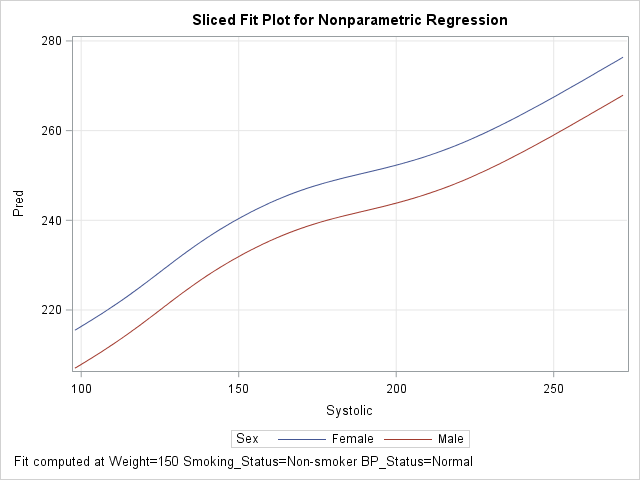The GAMPL procedure does not automatically include all input variables in the output data set; the ID statement specifies the variables that you want to output. The OUTPUT statement produces predicted values for all observations in the ALL data set, but the call to PROC SGPLOT creates the sliced plot by using only the observations for which ScoreData = 1. The output shows the nonparametric regression model from PROC GAMPL.

You can also use the ALL data set to overlay the original data and the sliced fit plot. The details are left as an exercise for the reader.

### Summary

The EFFECTPLOT statement provides an easy way to create a sliced fit plot. You can use the EFFECTPLOT statement directly in some regression procedures (such as LOGISTIC and GENMOD) or by using the STORE statement to save the model and PROC PLM to display the graph. For procedures that do not support the STORE statement, you can use the DATA step to create "the slice" (as a scoring data set) and use traditional scoring techniques to evaluate the model on the slice.

The post How to create a sliced fit plot in SAS appeared first on The DO Loop.Slice, slice, baby! You've got to slice, slice, baby!

When you fit a regression model that has multiple explanatory variables, it is a challenge to effectively visualize the predicted values. This article describes how to visualize the regression model by slicing the explanatory variables. In SAS, you can use the SLICEFIT option in the EFFECTPLOT statement visualize a slice of a regression surface.

### Why the naive visualization fails

For a regression model that contains one explanatory variable and (optionally) one classification variable, it is easy to visualize the predicted values. Most statistical software packages make it easy to create a "fit plot." For example, the following call to PROC GLM in SAS fits a model to some patients in a heart study:

```data Heart; /* create example data */ set sashelp.heart(obs=500); where cholesterol < 400; run;   ods graphics / attrpriority=none /* groups determine symbols and line patterns */ imagemap tipmax=1500; /* enable tool tips */   /* easy to visualize predicted values for 1 continuous and 1 categorical explanatory variable */ proc glm data=Heart plots=meanplot; /* PLOTS= option supported in many procedures */ class Sex; model Cholesterol = Sex Systolic; quit;```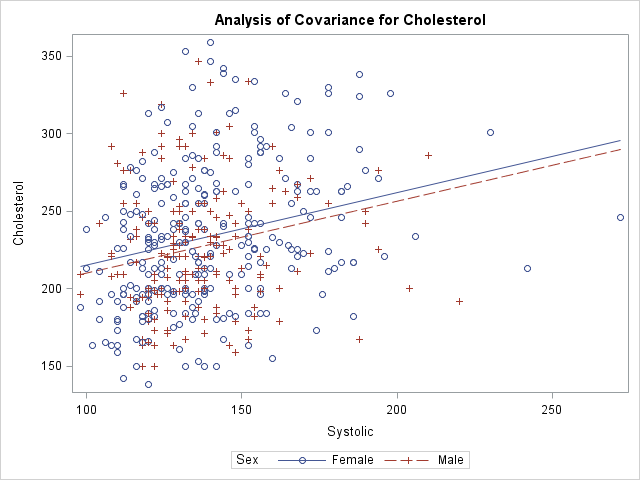The graph shows the observed responses versus the continuous explanatory variable and overlays two curves: one for the predicted values when Sex='Male' and the other when Sex='Female'. Creating this graph is easy because the procedure does all the work.

What happens if you add additional explanatory variables into the model and try to create the same graph? For reasons that will soon be apparent, the procedure will not automatically create the graph when there are additional variables in the model. However, you can use the OUTPUT statement to write the predicted values to a SAS data set and use PROC SGPLOT to create the graph. You will need to sort by the variable that you are plotting on the X axis, as follows:

```proc glm data=Heart; class Sex Smoking_status; model Cholesterol = Sex Smoking_Status /* two classification variables */ Systolic Weight; /* two continuous variables */ output out=GLMOut p=Pred; /* output data set contains predicted values */ quit;   proc sort data=GLMOut; by Systolic Sex; run; /* sort by X variable for graphing */   title "Predicted Values"; proc sgplot data=GLMOut; styleattrs datalinepatterns=(solid solid); scatter x=Systolic y=Cholesterol / group=Sex transparency=0.75; series x=Systolic y=Pred / group=Sex tip=(Smoking_Status Weight); /* add tool tips */ yaxis min=180 max=300; /* zoom in on predicted values */ footnote J=L "Jagged Lines Because Covariates Have Multiple Values"; run;```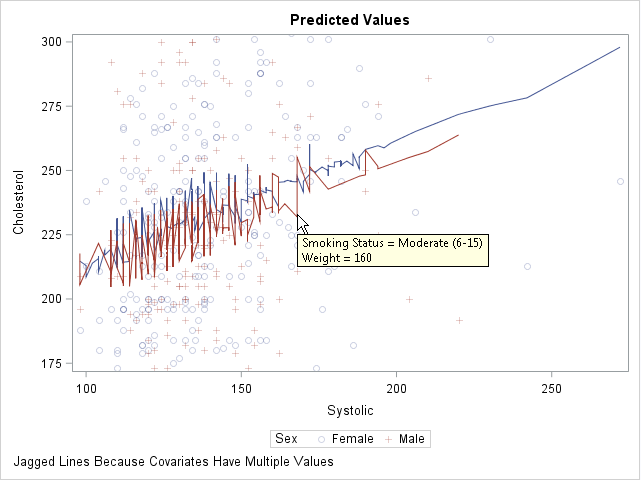This graph looks strange. The regression model is linear, but a plot of the predicted values shows a jagged line for the predicted values. What is going on?

You can use the tool tips feature of the graph to understand why the curves are jagged. If you hover the cursor near a point on the jagged line, the values of the hidden explanatory variables (Weight and Smoking_Status) appear. The graph shows the tool tip at a point that corresponds to a male patient who weighs 160 pounds and who is a moderate smoker. By moving the cursor, you can discover that the previous point along the red line corresponds to a male patient who weighs 155 pounds and is a non-smoker. The subsequent point corresponds to a heavy smoker who weighs 151 pounds.

Because Weight and Smoking_Status were included in the model, the predicted values "jump" up or down as you move along the Systolic axis. Two observations that have similar Systolic values might have very different values for other (hidden) components. Geometrically, this graph displays the projection of the predicted values onto the two-dimensional (Systolic, Cholesterol) plane. To obtain a smooth curve, you must "slice" a response surface rather than project it.

### Slice the response surfaces

The predicted values for this model form a set of 10 planes in the three-dimensional space (x, y, z) = (Systolic, Weight, Cholesterol). Each plane is the graph of predicted values for a combination of the 2 genders and 5 levels of smokers. There is one plane is for the ('Male', 'Non-smoker') patients, another for the ('Female', 'Light (1-5)') patients, and so on.

A "slice" through the response surfaces is accomplished by evaluating the model at a particular value of one of the continuous variables. This gives a two-dimensional plot that has 10 lines on it. Because 10 lines might overcrowd the display, it is common to pick a reference value for one of the classification variables and plot only the lines that are indexed by that value. For example, if you choose the reference value Smoking_Status = 'Non-smoker', the plot contains two lines that correspond to ('Male', 'Non-smoker') and ('Female', 'Non-smoker').

This might sound complicated, but SAS provides an easy implementation: the SLICEFIT option in the EFFECTPLOT statement, which is supported in several regression procedures, enables you to specify how you want to slice the surfaces and which combinations of levels you want to display.

By default, the EFFECTPLOT SLICEFIT statement creates a "sliced fit plot" that graphs the response variable versus the first continuous variable and shows the predicted values for each level of the first class variable. "First" is determined by the order in which the variables are listed on the MODEL statement. Other continuous variables are sliced (evaluated) at their mean value; other classification variables are evaluated at their last level.

PROC GLM does not support the EFFECTPLOT statement, but PROC GENMOD does. The following call to PROC GENMOD fits the same model and creates a "sliced fit plot" of the predicted values. The sliced fit plot will show the response variable (Cholesterol) versus the first continuous variable (Systolic) overlaid with predictions for males and females. The value of the Weight variable is set to 151.7, which is the mean value of the sample. The value of the Smoking_Status variable is set to 'Very Heavy (> 25)', which is the last level in alphanumeric order.

```title; footnote; ods graphics / attrpriority=none imagemap=off; proc genmod data=Heart; class Sex Smoking_status; model Cholesterol = Sex Smoking_Status /* classification variables */ Systolic Weight; /* continuous variables */ /* Plot response vs first cont var for each level of first class var */ /* Set other cont vars to MEAN; set other class vars to last level */ effectplot slicefit / obs; /* add scatter plot of observations */ run;```The sliced fit plot shows smooth (not jagged) lines because the model is evaluated at constant values of the hidden variables. The values (Weight, Smoking_Status) = (151.7, 'Very Heavy (> 25)') are held constant while the model is evaluated over the range of the Systolic and Sex variables.

### Other ways to slice the response surfaces

The SLICEFIT option in the EFFECTPLOT statement supports many suboptions that enable you to control the way that the model is sliced:

• You can plot any two variables, one continuous and one categorical. Use the X= option to specify the continuous variable and the SLICEBY= option to specify the categorical variable.
• You can specify the statistics that are used to slice the continuous covariates. By default the covariates are sliced at their mean values. You can use the AT option to specify the following keywords: MEAN (the default), MIN, MAX, MEDIAN, or MIDRANGE. (Recall that the midrange is the value (min+max)/2.) For class variables, the REF option specifies that the last level be used.
• You can use the AT option to specify particular values for slicing the continuous covariates and class variables.
• You can specify multiple values for the AT option. The EFFECTPLOT statement will create a panel of sliced fit plots, one for each joint combination of specified values.

The following four EFFECTPLOT statements correspond to the four items in the previous list:

```proc genmod data=Heart; class Sex Smoking_status; model Cholesterol = Sex Smoking_Status /* classification variables */ Systolic Weight; /* continuous variables */ /* specify the X and categorical variables */ effectplot slicefit(X=weight sliceby=Smoking_status) / obs;   /* specify statistics used to slice the covariates */ effectplot slicefit / at MIDRANGE /* new default for continuous vars */ REF; /* default for classification vars */   /* specify explicit values of the covariates */ effectplot slicefit / at(Weight=150 Smoking_Status='Non-smoker');   /* specify multiple values of the covariates to get a panel */ effectplot slicefit / at(Weight=150 200 Smoking_Status='Non-smoker' 'Heavy (16-25)'); quit;```

To save space, only the last sliced fit plot (the panel) is shown below. I have linked to the other three plots: the plot of Weight and Smoking_Status, the plot at midrange, and the plot at specified values.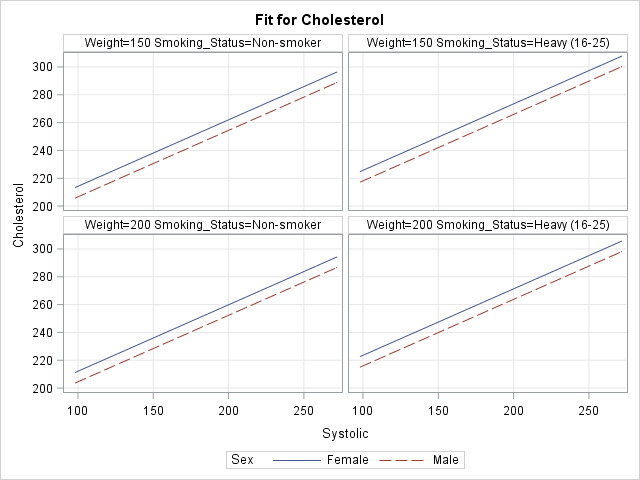In summary, you can use the SLICEFIT option in the EFFECTPLOT statement in SAS to visualize regression models that contain many explanatory variables. The AT option enables you to specify values for the covariates. The resulting graph displays a slice through the response surface.

The EFFECTPLOT statement is also available in PROC PLM. PROC PLM enables you to visualize a model that has been saved to an item store. The OBS option (which overlays the predicted values and a scatter plot) is not available in PROC PLM because the item store does not include the observations.

The post Visualize multivariate regression models by slicing continuous variables appeared first on The DO Loop.In a previous article, I showed how to use SAS to perform mean imputation. However, there are three problems with using mean-imputed variables in statistical analyses:

• Mean imputation reduces the variance of the imputed variables.
• Mean imputation shrinks standard errors, which invalidates most hypothesis tests and the calculation of confidence interval.
• Mean imputation does not preserve relationships between variables such as correlations.

This article explores these issues in more detail. The example data set (called IMPUTED) was created in the previous article. It is a modification of the Sashelp.Class data in which heights of seven students are assigned missing value. Mean imputation replaces those seven value with the mean of the observed values. The Orig_Height variable contains the original (missing) values; the Height variable contains the imputed values.

### Mean imputation reduces variance

The following call to PROC MEANS computes simple descriptive statistics for the original and imputed variables. The statistics for the original variable are computed by using listwise deletion, which means that missing observations are dropped from the analysis.

```proc means data=Imputed ndec=2 N NMiss Mean StdErr StdDev; var Orig_Height Height; /* compare stats original and imputed vars */ run;```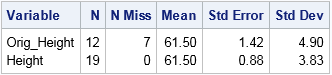The mean-imputed variable (Height) has the same mean as the original variable (Orig_Height). This is always the case for mean-imputed data. However, notice that the standard deviation (hence, variance) of the imputed variable is smaller. You can see this by overlaying the distributions of the original and imputed variables, as follows:

```title "Comparison of Original and Imputed Data"; proc sgplot data=Imputed; xaxis label="Height" values=(52 to 70 by 4 61.5) valueshint; yaxis grid; histogram Height / scale=count binstart=52 binwidth=4 legendlabel="Imputed"; histogram Orig_Height / scale=count binstart=52 binwidth=4 legendlabel="Original" transparency=0.5; refline 61.5 / axis=x lineattrs=(color=black) label="Mean"; /* mean value */ run;```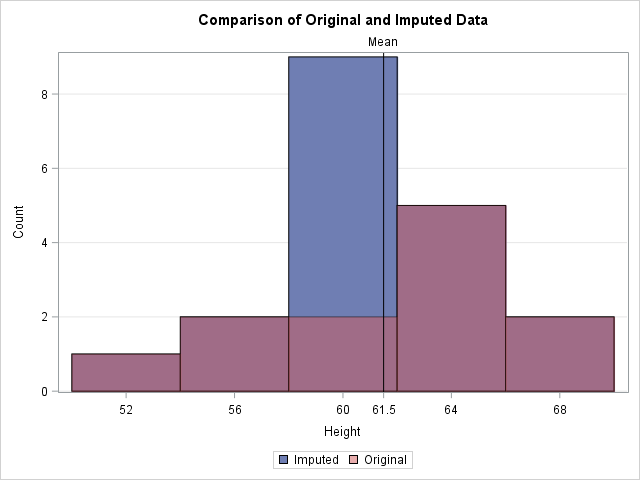In the graph, the reddish bars show the distribution of the observed values. Behind those bars is a second histogram (in blue) that shows the distribution of the imputed data. The only bar of the second histogram that is visible is the one that contains the sample mean. The graph emphasizes the fact that all imputed values are equal to the mean. This reduces the variance of the imputed variable because none of the imputed values contribute to the variance (which is based on deviations from the mean). Thus the variance of the mean-imputed variable is always smaller than the variance of the original variable.

### Mean imputation shrinks standard errors and confidence intervals

The previous section shows that the imputed variable always has a smaller variance than original variable. The estimated variance is used to compute many other statistics, which are also shrunk. For example, the following statistics are shrunk for the imputed variable as compared to the original variable:

1. The standard error of the mean, as shown in the previous output from PROC MEANS.
2. The confidence intervals that are based on mean-imputed data will be shorter.
3. The standard t test for a mean uses the standard error to compute a p-value for the null hypothesis that the population mean equals some value. If the standard error is shrunk by mean imputation, then the standard one-sample t test is not valid and the p-value is too small. You will potentially reject a null hypotheses that might be true (a Type I error).

### Mean imputation distorts relationships between variables

The previous sections emphasized how mean imputation affects univariate statistics. But mean imputation also distorts multivariate relationships and affects statistics such as correlation. For example, the following call to PROC CORR computes the correlation between the Orig_Height variable and the Weight and Age variables. It also computes the correlations for the mean-imputed variable (Height). The output shows that the imputed variable has much smaller correlations with Weight and Age because single imputation does not try to preserve multivariate relationships.

```proc corr data=Imputed noprob; var Weight Age; with Orig_Height Height; run;```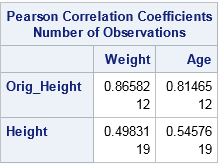In a similar way, a linear regression that attempts to predict Weight by height is corrupted by the replacement of missing values with mean values. For one-variable linear regression, it is easy to show that the estimates of the slope are unchanged by mean imputation, but the intercept estimates can be different. For these data, the least-squares estimate of the slope is 2.96. The intercept estimate for the original data is -90 whereas the intercept for the imputed variable is -82. The following call to PROC SGPLOT shows these estimates graphically:

```ods graphics / attrpriority=NONE; title "Simple Linear Regression with Mean Imputation"; proc sgplot data=Imputed; styleattrs datasymbols=(Circle X); reg x=Orig_Height y=Weight / nomarkers curvelabel="Original"; reg x=Height y=Weight / nomarkers curvelabel="Imputed"; scatter x=Height y=Weight / group=Replaced; xaxis grid; yaxis grid; run;```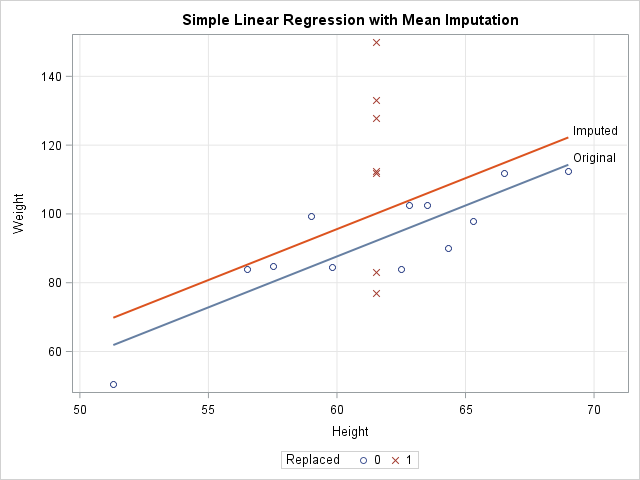The graph shows that the model that uses the original data (the blue line) predicts lower values of Weight than the model that uses the imputed heights (the red line). The scatter plot shows why. The model for the original data uses only 12 observations, which are displayed as blue circles. The predicted value of Weight for the mean height is about 92. The seven imputed values are shown as red X's for which the Height is 61.5. The average Weight for these observations is greater than 92, so the seven observations bias the computation and "pull up" the regression line. For different data, the imputed model might "pull down" the predictions. It depends on the average response for the imputed observations.

### Conclusions

Although imputing missing values by using the mean is a popular imputation technique, there are serious problems with mean imputation. The variance of a mean-imputed variable is always biased downward from the variance of the un-imputed variable. This bias affects standard errors, confidence intervals, and other inferential statistics. Experts agree that mean imputation should be avoided when possible (Allison (2009), Horton and Kleinman (2007)).

So what alternatives are there? That question has been the topic of many books and papers. Paul Allison (2009) suggests either maximum likelihood estimation or multiple imputation methods, both of which try to preserve relationships between variables and the inherent variability of the data. In SAS, PROC MI and MIANALYZE work with other SAS/STAT procedures to apply these methods to missing data. You can see the list of procedures that handle missing data in SAS. For more information about the alternatives to single imputation, the following references are good places to start:

The post 3 problems with mean imputation appeared first on The DO Loop.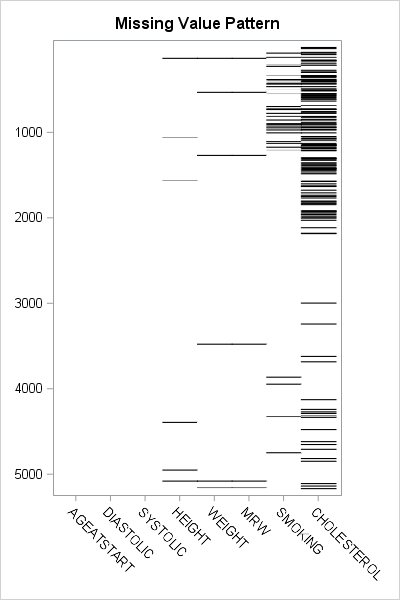Missing values present challenges for the statistical analyst and data scientist. Many modeling techniques (such as regression) exclude observations that contain missing values, which can reduce the sample size and reduce the power of a statistical analysis. Before you try to deal with missing values in an analysis (for example, by using multiple imputation), you need to understand which variables contain the missing values and to examine the patterns of missing values. I have previously written about how to use SAS to do the following:

An example of a visualization of a missing value pattern is shown to the right. The heat map shows the missing data for eight variables and 5209 observations in the Sashelp.Heart data set. It is essentially the heat map of a binary matrix where 1 indicates nonmissing values (white) and 0 indicates missing values (black).

In this article, I present a bar chart that helps to visualize the "Missing Data Patterns" table from PROC MI in SAS/STAT software. I also show two SAS tricks:

### The pattern of missing data

The MI procedure can perform multiple imputation of missing data, but it also can be used as a diagnostic tool to group observations according to the pattern of missing data. You can use the NIMPUTE=0 option to display the pattern of missing value. By default, the "Missing Data Patterns" table is very wide because it includes the group means for each variable. However, you can use the DISPLAYPATTERN=NOMEANS option (SAS9.4M5) to suppress the group means, as follows:

```%let Vars = AgeAtStart Height Weight Diastolic Systolic MRW Smoking Cholesterol; %let numVars = %sysfunc(countw(&Vars)); /* &numVars = 8 for this example */   proc mi data=Sashelp.Heart nimpute=0 displaypattern=nomeans; /* SAS 9.4M5 option */ var &Vars; ods output MissPattern=Pattern; /* output missing value pattern to data set */ run;```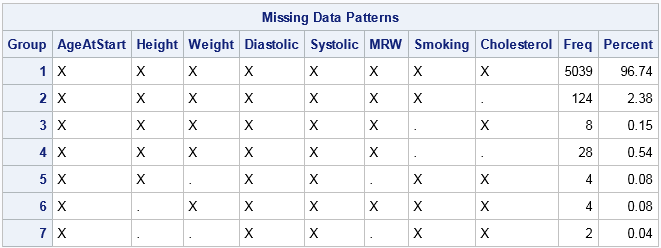In the table, an X indicates that a variable does not contain any missing values, whereas a dot (.) indicates that a variable contains missing values. The table shows that Group 1 consists of about 5000 observations that are complete. Groups 2, 3, and 6 contains observations for which exactly one variable contains missing values. Groups 4 and 5 contain observations for which two variables are jointly missing. Group 7 contains observations for which three variables are missing. You can see that the size of the groups vary widely. Some patterns of missing value appear only a few times, whereas others appear much more often.

By inspecting the table, you can determine which combinations of variables are missing for each group. However, imagine a dataset that has 20 variables. The "Missing Data Patterns" table for 20 variables would be very wide, and it would be cumbersome to determine which combination of variables are jointly missing. The next section condenses the table into a smaller format and then creates a graph to summarize the pattern of missing values.

### Shorten the labels for the missing data patterns

The information in the "Missing Data Patterns" table can be condensed. I saw the following idea in Chapter 10 of Gerhard Svolba's Data Quality for Analytics Using SAS, who credits the idea to a display that appears in JMP software. (Svolba does not use PROC MI, but defines a macro that you can download from the book's website.)

The table is wide because there is a column for every variable in the analysis. But the information in those columns is binary: for the i_th group does the j_th variable have a missing value? If the analysis involves k variables, you can replace the k columns by a single binary string that has k digits. The j_th digit in the string indicates whether the j_th variable has a missing value.

In the preceding analysis, the ODS OUTPUT statement wrote the "Missing Data Patterns" table to a data set. The following SAS DATA step reads the data and constructs a binary string of length k from the k character variables in the data. The binary string is formed by using the CATT function to concatenate the 'X' and '.' values in the table. The TRANSLATE function then converts those characters to '0' and '1' characters. The DATA step also computes the NumMiss variable, which counts the number of variables that have missing values for each row:

```data Miss; set Pattern; array vars[*] _CHARACTER_; /* or use &Vars */ length Pattern \$&numVars.; /* length = number of variables in array */ Pattern = translate(catt(of vars[*]), '01', 'X.'); /* Ex: 00100100 */ NumMiss = countc(pattern, '1'); run;   proc print data=Miss noobs; var Group Pattern NumMiss Freq; run;```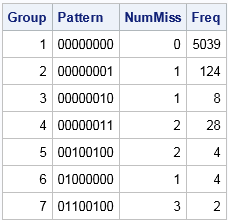The table shows that the eight columns for the analysis variables have been replaced by a single column that displays an eight-character binary string. For Group 1, the string is all zeros, which indicates that no variable has missing values. For Group 2, the binary string contains all zeros except for a 1 in the last position. This means that Group 1 is the set of observations for which the last variable contains a missing value. Similarly, Group 7 is the set of observations for which the second, third, and sixth variables contain a missing value. Notice that this table does not provide the names of the variables. If you cannot remember the name of the second, third, and sixth variables, you need to look them up in the VARS macro variable.

### Create a bar chart that has a logarithmic scale

The condensed version of the "Missing Data Patterns" table is suitable to graph as a bar chart. However, for these data the size of the groups vary widely. Consequently, you might want to plot the frequencies of the groups on a logarithmic scale. (Or you might not! There is considerable debate about whether you should display a bar chart that has a logarithmic axis. For a discussion and alternatives, see Sanjay's article "Graphs with log axis." My opinion is that a log axis is fine to use for a technical audience such as statisticians.)

By default, you cannot use a logarithmic scale on a bar chart because the baseline for the vertical axis (the frequency or count axis) starts at 0 and the LOG function is not defined at 0. If you have count data and no category has zero counts, then you can set the baseline of the graph to 1. The bars then indicate the log-frequency of the counts. The following call to PROC SGPLOT creates the bar chart and a marginal table:

```title "Pattern of Missing Values"; proc sgplot data=Miss; hbar Pattern /response=Freq datalabel baseline=1; /* set BASELINE=1 for log scale */ yaxistable NumMiss / valuejustify=left label="Num Miss" valueattrs=GraphValueText labelattrs=GraphLabelText; /* use same attributes as axis */ yaxis labelposition=top; xaxis grid type=log logbase=10 label="Frequency (log10 scale)"; run;```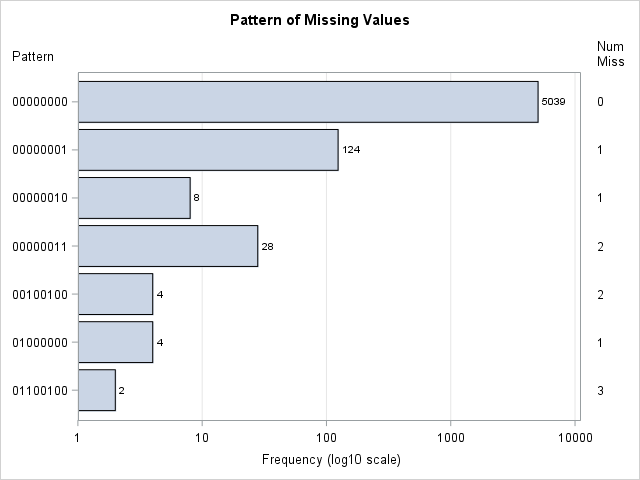This graph displays the counts of the number of observations in each pattern group. The labels on the Y axis indicate which variables have missing data. The values in the right margin indicate how many variables have missing data. If you are opposed to drawing bar charts on a logarithmic axis, you can use one of Sanjay's alternative visualizations.

In summary, you can create a bar chart that visualizes the number of observations that have a similar pattern of missing values. The summarization comes from PROC MI in SAS, which has a new DISPLAYPATTERN=NOMEANS option. A short DATA step can create a binary string for each group. That string can be used to indicate the missing data pattern in each group. If the groups vary widely in size, you can use a logarithmic axis to display the data. To create a bar chart on a logarithmic scale in SAS, set BASELINE=1 in the HBAR statement and use TYPE=LOG on the XAXIS statement in PROC SGPLOT.

The post Visualize patterns of missing values appeared first on The DO Loop.Missing values present challenges for the statistical analyst and data scientist. Many modeling techniques (such as regression) exclude observations that contain missing values, which can reduce the sample size and reduce the power of a statistical analysis. Before you try to deal with missing values in an analysis (for example, by using multiple imputation), you need to understand which variables contain the missing values and to examine the patterns of missing values. I have previously written about how to use SAS to do the following:

An example of a visualization of a missing value pattern is shown to the right. The heat map shows the missing data for eight variables and 5209 observations in the Sashelp.Heart data set. It is essentially the heat map of a binary matrix where 1 indicates nonmissing values (white) and 0 indicates missing values (black).

In this article, I present a bar chart that helps to visualize the "Missing Data Patterns" table from PROC MI in SAS/STAT software. I also show two SAS tricks:

### The pattern of missing data

The MI procedure can perform multiple imputation of missing data, but it also can be used as a diagnostic tool to group observations according to the pattern of missing data. You can use the NIMPUTE=0 option to display the pattern of missing value. By default, the "Missing Data Patterns" table is very wide because it includes the group means for each variable. However, you can use the DISPLAYPATTERN=NOMEANS option (SAS9.4M5) to suppress the group means, as follows:

```%let Vars = AgeAtStart Height Weight Diastolic Systolic MRW Smoking Cholesterol; %let numVars = %sysfunc(countw(&Vars)); /* &numVars = 8 for this example */   proc mi data=Sashelp.Heart nimpute=0 displaypattern=nomeans; /* SAS 9.4M5 option */ var &Vars; ods output MissPattern=Pattern; /* output missing value pattern to data set */ run;```In the table, an X indicates that a variable does not contain any missing values, whereas a dot (.) indicates that a variable contains missing values. The table shows that Group 1 consists of about 5000 observations that are complete. Groups 2, 3, and 6 contains observations for which exactly one variable contains missing values. Groups 4 and 5 contain observations for which two variables are jointly missing. Group 7 contains observations for which three variables are missing. You can see that the size of the groups vary widely. Some patterns of missing value appear only a few times, whereas others appear much more often.

By inspecting the table, you can determine which combinations of variables are missing for each group. However, imagine a dataset that has 20 variables. The "Missing Data Patterns" table for 20 variables would be very wide, and it would be cumbersome to determine which combination of variables are jointly missing. The next section condenses the table into a smaller format and then creates a graph to summarize the pattern of missing values.

### Shorten the labels for the missing data patterns

The information in the "Missing Data Patterns" table can be condensed. I saw the following idea in Chapter 10 of Gerhard Svolba's Data Quality for Analytics Using SAS, who credits the idea to a display that appears in JMP software. (Svolba does not use PROC MI, but defines a macro that you can download from the book's website.)

The table is wide because there is a column for every variable in the analysis. But the information in those columns is binary: for the i_th group does the j_th variable have a missing value? If the analysis involves k variables, you can replace the k columns by a single binary string that has k digits. The j_th digit in the string indicates whether the j_th variable has a missing value.

In the preceding analysis, the ODS OUTPUT statement wrote the "Missing Data Patterns" table to a data set. The following SAS DATA step reads the data and constructs a binary string of length k from the k character variables in the data. The binary string is formed by using the CATT function to concatenate the 'X' and '.' values in the table. The TRANSLATE function then converts those characters to '0' and '1' characters. The DATA step also computes the NumMiss variable, which counts the number of variables that have missing values for each row:

```data Miss; set Pattern; array vars[*] _CHARACTER_; /* or use &Vars */ length Pattern \$&numVars.; /* length = number of variables in array */ Pattern = translate(catt(of vars[*]), '01', 'X.'); /* Ex: 00100100 */ NumMiss = countc(pattern, '1'); run;   proc print data=Miss noobs; var Group Pattern NumMiss Freq; run;```The table shows that the eight columns for the analysis variables have been replaced by a single column that displays an eight-character binary string. For Group 1, the string is all zeros, which indicates that no variable has missing values. For Group 2, the binary string contains all zeros except for a 1 in the last position. This means that Group 1 is the set of observations for which the last variable contains a missing value. Similarly, Group 7 is the set of observations for which the second, third, and sixth variables contain a missing value. Notice that this table does not provide the names of the variables. If you cannot remember the name of the second, third, and sixth variables, you need to look them up in the VARS macro variable.

### Create a bar chart that has a logarithmic scale

The condensed version of the "Missing Data Patterns" table is suitable to graph as a bar chart. However, for these data the size of the groups vary widely. Consequently, you might want to plot the frequencies of the groups on a logarithmic scale. (Or you might not! There is considerable debate about whether you should display a bar chart that has a logarithmic axis. For a discussion and alternatives, see Sanjay's article "Graphs with log axis." My opinion is that a log axis is fine to use for a technical audience such as statisticians.)

By default, you cannot use a logarithmic scale on a bar chart because the baseline for the vertical axis (the frequency or count axis) starts at 0 and the LOG function is not defined at 0. If you have count data and no category has zero counts, then you can set the baseline of the graph to 1. The bars then indicate the log-frequency of the counts. The following call to PROC SGPLOT creates the bar chart and a marginal table:

```title "Pattern of Missing Values"; proc sgplot data=Miss; hbar Pattern /response=Freq datalabel baseline=1; /* set BASELINE=1 for log scale */ yaxistable NumMiss / valuejustify=left label="Num Miss" valueattrs=GraphValueText labelattrs=GraphLabelText; /* use same attributes as axis */ yaxis labelposition=top; xaxis grid type=log logbase=10 label="Frequency (log10 scale)"; run;```This graph displays the counts of the number of observations in each pattern group. The labels on the Y axis indicate which variables have missing data. The values in the right margin indicate how many variables have missing data. If you are opposed to drawing bar charts on a logarithmic axis, you can use one of Sanjay's alternative visualizations.

In summary, you can create a bar chart that visualizes the number of observations that have a similar pattern of missing values. The summarization comes from PROC MI in SAS, which has a new DISPLAYPATTERN=NOMEANS option. A short DATA step can create a binary string for each group. That string can be used to indicate the missing data pattern in each group. If the groups vary widely in size, you can use a logarithmic axis to display the data. To create a bar chart on a logarithmic scale in SAS, set BASELINE=1 in the HBAR statement and use TYPE=LOG on the XAXIS statement in PROC SGPLOT.

The post Visualize patterns of missing values appeared first on The DO Loop.